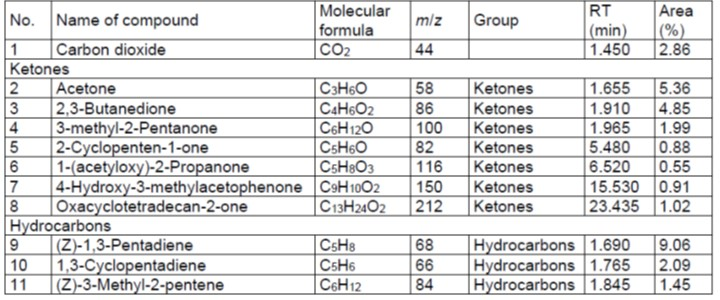Xu, L., Zhang, Y., Wang, Z., Guo, S., Hao, Y., Gao, Y., Xin, M., Ran, Y., Li, S., Ji, R., Li, H., Jiang, H., He, Q., and Huang, R. (2023). “Kinetic analysis and pyrolysis behavior of pine needles by TG-FTIR and Py-GC/MS,” BioResources 18(3), 6412-6429.

#### Abstract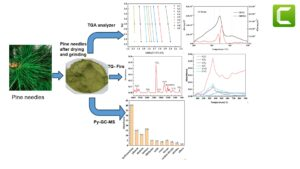The pyrolysis performances and reaction kinetics of pine needles (PN) were investigated by integrating thermogravimetric analysis, Fourier transform infrared spectroscopy, and pyrolysis-gas chromatography/mass spectrometry. The average activation energy of PN was estimated to be 183.2 kJ/mol by Kissinger Akahira Sunose (KAS) and 183.8 kJ/mol by Flynn Wall Ozawa (FWO), respectively at heating rates of 10, 20, and 40 °C/min. The pyrolysis of PN was found to be more efficient at the lower heating rates, while increased heating rates promoted the reaction. Using the King-Kai (K-K) method, the activation energies of hemicellulose, cellulose, and lignin were calculated to be 156, 165, and 172 kJ/mol, respectively. The descending order of evolving gases and functional groups from PN was found to be CO2, C=C, C=O, H2O, CH4, and CO. The main pyrolytic by-products identified were hydrocarbons, phenols, alcohols, ketones, and aldehydes. The determination of kinetic parameters provides fundamental information for predicting the rates at which chemical reactions occur. This study demonstrates the potential of PN as a suitable source for bioenergy.

Kinetic Analysis and Pyrolysis Behavior of Pine Needles by TG-FTIR and Py-GC/MS

Langui Xu,a Yujian Zhang,a Ziyong Wang,b Shurui Guo,c Yongxing Hao,a Yuguo Gao,a Min Xin,d Yi Ran,e Shuxun Li,f Rui Ji,g Hongmei Li,g Huixia Jiang,h Qingyan He,c,h and Ruyi Huang a,d,e,*

The pyrolysis performances and reaction kinetics of pine needles (PN) were investigated by integrating thermogravimetric analysis, Fourier transform infrared spectroscopy, and pyrolysis-gas chromatography/mass spectrometry. The average activation energy of PN was estimated to be 183.2 kJ/mol by Kissinger Akahira Sunose (KAS) and 183.8 kJ/mol by Flynn Wall Ozawa (FWO), respectively at heating rates of 10, 20, and 40 °C/min. The pyrolysis of PN was found to be more efficient at the lower heating rates, while increased heating rates promoted the reaction. Using the King-Kai (K-K) method, the activation energies of hemicellulose, cellulose, and lignin were calculated to be 156, 165, and 172 kJ/mol, respectively. The descending order of evolving gases and functional groups from PN was found to be CO2, C=C, C=O, H2O, CH4, and CO. The main pyrolytic by-products identified were hydrocarbons, phenols, alcohols, ketones, and aldehydes. The determination of kinetic parameters provides fundamental information for predicting the rates at which chemical reactions occur. This study demonstrates the potential of PN as a suitable source for bioenergy.

DOI: 10.15376/biores.18.3.6412-6429

Keywords: Pine needle; Pyrolysis; Thermogravimetric analysis; TG–FTIR; Py–GC/MS

Contact information: a: School of Mechanical Engineering, North China University of Water Resources and Electric Power, Zhengzhou 450011, China; b: Henan ALST New Energy Technology Co. LTD, Zhengzhou 450001, China; c: College of Architecture and Environment, Sichuan University, Chengdu China, 610065; d: Rural Energy Development Center of Sichuan Province, Chengdu 610041, China; e: Biogas Institute of Ministry of Agriculture and Rural Affairs, Key Laboratory of Development and Application of Rural Renewable Energy, Ministry of Agriculture and Rural Affairs, Chengdu 610041, China; f: Sichuan Water Conservancy Vocational and Technical College, Chengdu 611830, China; g: Sichuan Agricultural Foreign Cooperation and Communication Center Chengdu 610041, China; h: Sichuan Research and Design Institute of Agricultural Machinery Chengdu 610066, China;

* Corresponding author: 28209443@qq.com

GRAPHICAL ABSTRACTINTRODUCTION

Biomass is an important alternative energy source due to its sustainability, carbon neutrality, and abundance (Wang et al. 2018; Huang et al. 2020). Through thermochemical conversion technology, energy recycling can be achieved (Ding et al. 2020). Forest residues have received extensive consideration as biofeedstock due to their wide geographical distribution, lower emissions released into the environment, and high biomass potential (Dhyani and Bhaskar 2018). Bioenergy is projected to rank as the fourth energy resource with a projected growth rate of 30%, following oil, coal, and natural gas (Yasmeen et al. 2022). Pine is a gymnosperm belonging to the Pinaceae family, and its leaves are needle-shaped, so it is named after “pine needle” (PN). There are rich PN resources in China, with reserves of more than 100 million tons every year (Wang et al. 2020). Transforming PN into biofuel through pyrolysis and combustion can offer a comprehensive and sustainable solution to multiple issues. The accumulation of pine needles can pose challenges for local residents, including the risk of wildfires and environmental concerns.

During biomass combustion, the process typically starts with the pyrolysis stage. Pyrolysis is the thermal decomposition of biomass with limited oxygen supply. Under external heat, biomass undergoes several stages of pyrolysis, which can convert raw materials into bio-oil, bio-char, and pyrolytic gases (Gupta et al. 2019). For example, Fu et al. (2022) found that the pyrolysis of torrefied coffee grounds at 300 °C reduced the hydrocarbon proportion of bio-oils. According to Huang et al. (2020), the main pyrolytic products obtained from water hyacinth roots, stems, and leaves were phenols, furans, and nitrides. Pine trunks have been investigated in related literature, indicating their pyrolysis characteristics with the exclusion of their residues such as PN (Sun et al. 2017; Xu et al. 2020). The pyrolysis conditions (such as temperature, heating rate) and their impact on the yield of bio-oil obtained from pine needles have been studied (Varmal et al. 2015, 2018). These studies aim to optimize the process parameters to maximize the bio-oil yield and improve its quality. Catalysts can enhance the pyrolysis process by promoting specific reactions and improving the yield and quality of the resulting bio-oil. The effects of catalysts on the pyrolysis kinetic parameters of pine needles and the composition of bio-oil were explored (Gupta and Mondal 2021). The pyrolytic gases can contribute to the combustion process and energy generation when pine needles are burned. Researching the pyrolysis characteristics of pine needles, analyzing the composition of pyrolysis products, and exploring ways to enhance the production of pyrolysis gases will contribute to achieving complete combustion of pine needles. The distribution and composition of the products obtained from pyrolysis depend on various factors, such as temperature, heating rate, residence time, biomass feedstock, and pyrolysis process conditions. Optimization of these parameters can help tailor the pyrolysis process to maximize the desired product yields or specific properties of the products, such as higher gas production.

The pyrolysis of biomass is a complex process involving various thermochemical reactions such as char formation, fragmentation, depolymerization, and secondary mechanisms (Collard and Blin 2014). The evaluation of kinetic parameters is essential for understanding and predicting the dynamic conversion of biomass (Wang et al. 2017). Thermogravimetric analysis (TGA) is a commonly used method for characterizing the pyrolysis process and kinetics of various feedstocks (Tang et al. 2020; Ding et al. 2022).

The integration of kinetic and thermodynamic analyses using TG data is vital for estimating activation energy, designing and managing pyrolysis processes, acquiring pyrolytic byproducts, and facilitating scale-up for industrial applications. Monitoring the evolved products from PN pyrolysis can be effectively achieved by combining thermogravimetric analysis (TGA) with thermogravimetric analysis coupled with Fourier transform infrared spectroscopy (TG-FTIR) (Mehmood et al. 2019; Huang et al. 2020). Pyrolysis-gas chromatography/mass spectrometry (Py-GC/MS) is a technique commonly used to quantitatively investigate the product distribution from biomass pyrolysis with NIST library (Li et al. 2021; Nardella et al. 2022). In turn, this can provide insights for optimizing the distribution of pyrolysis products to enhance combustion efficiency.

In the present study, the thermogravimetric analysis of PN at the three heating rates (10, 20, and 40 °C/min) was carried out, and the kinetic and thermodynamic parameters were estimated. The pyrolysis mechanism of three components of PN (hemicellulose, cellulose, and lignin) was studied. The gas emissions and pyrolytic products were determined by TG-FTIR and Py-GC/MS. The results provide guidance for the effective utilization of PN.

EXPERIMENTAL

Sample Pretreatment

The PN sample used in this study was collected from the countryside of Nanyang in Henan province of China. The samples were crushed and screened through 80-mesh sieve, after which they were dried at 80 °C for 24 h to remove moisture.

TG Experiments

The thermogravimetric experiment was conducted using an SDT Q600 TG analyzer from America, under a N2 atmosphere. Samples weighing approximately 8.0 ± 0.5 mg were placed in Al2O3 crucibles. The TG experiments were heated from room temperature to 900 °C at heating rates of 10, 20, and 40 °C/min, with a nitrogen gas flow rate of 100 mL/min.

Reaction Kinetics of Pyrolysis

The TG results can be used to calculate kinetic parameters of biomass (Siddiqi et al. 2020). The pyrolysis reactions can be expressed as Eq. 1,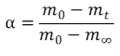(1)

where α is the conversion degree at time t (s), m0 is the mass of initial time, mt is the real-time mass at time t, and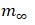is the remaining mass at the maximum pyrolysis temperature. The rate of mass change can be expressed as Eq. 2,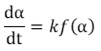(2)

where f(α) is the conversion function, which can be defined as Eq. 3: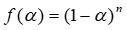(3)

The PN pyrolysis was assumed to follow the first-order reaction model, where n = 1. In Eq. 2, k is the reaction constant, which can be expressed as Eq. 4,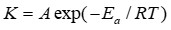(4)

where A is the pre-exponential factor, Eα denotes the activation energy, R is the universal gas constant (8.31 J/(K·mol)), and T is the reaction temperature (°C).

The heating rate is constant and defined as Eq. (5):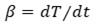(5)

By combining equations 1, 3, 4, and 5, Eq. 6 can be obtained: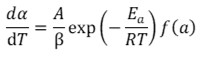(6)

The integral function of conversion rate is assumed as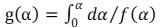, such that the equation can be expressed as follows (Eq. 7),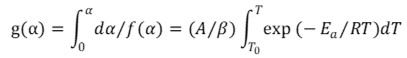(7)

In this study, the model-free methods of KAS and KWO method were adopted to estimate the Eα values of PN.

KAS method

KAS method was performed as shown by Kissinger et al. (1957) and Akahira and Sunose (1971). The key relationship can be expressed as Eq. 8,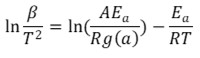(8)

For a certain constant g(α), the relationship between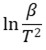and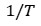is a straight line. Eα was calculated with the line slope of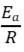. A can be estimated by the line intercept.

FWO method

The FWO method is based on Doyle’s approximation and the expression as following,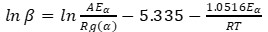(9)

For a certain constant g(a), the line between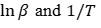is also a straight line. Eα is estimated with the slope expression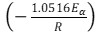.

Kissinger – Kai method

The K-K method was developed from the Kissinger method (Kissinger et al. 1956; Li et al. 2014). The second derivative maxima of TG correspond to the peak locations for hemicellulose, cellulose and lignin, the expression as Eq. 10,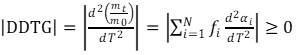(10)

where N is the total number of constituents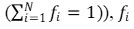is the mass fraction of every constituent (i). As one constituent approaches the peak of reaction speed, the second derivative drops to a minimum. The activation energies for constituents are obtained as Eq. 11,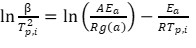(11)

where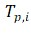is the peak temperature of every constituent, the line between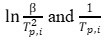is plotted, and Eα of every component can be calculated from the slope expression.

TG–FTIR Experiments

Thermogravimetry (TGA 209 F3, Netzsch, Germany) together with a FTIR spectrometer (Bruker IFS 66/S, Bruker Optics, Billerica, MA) was used to analyze the evolved gases of PN pyrolysis. Samples of 8 ± 0.5 mg were placed in TG reactor and heated from room temperature to 900 °C with a rate of 10 °C/min, while the gas flow rate with the nitrogen was set as 70 mL/min. The temperature of the transmission line between TG and FTIR was set to 210 ℃ to prevent the produced gases condensation. The scanning resolution of FTIR was set to 8 cm-1 in the range of 600 to 4000 cm-1, and data collection time of 60 min.

Py–GC/MS Experiments

A Py–GC/MS experiment was conducted to identify the pyrolysis volatiles using a Frontier pyrolyzer (EGA/PY-3030D, Japan) coupled to a GC/MS equipment (GCMS-QP 2010 Ultra, Shimadzu) equipped with a quartz capillary column (30 m × 0.25 mm × 0.25 μm). For each sample (0.10 mg), a vertical quartz tube was utilized, and silica wool was employed to prevent the escape of solid particles. Pyrolysis of the samples was performed at 600 °C for 10 seconds. The GC injector temperature was set to 250 °C, while the temperature of the chromatographic column was programmed as follows: (i) 50 °C for 5 minutes, (ii) ramping from 50 °C to 260 °C at a rate of 10 °C/min, and (iii) maintaining the temperature at 260 °C for 10 min. The carrier gas (helium) flow rate was set to 1.27 mL/min with a split ratio of 100:1. Mass spectra in total ion current mode were obtained within the mass range of m/z 35-500. The yield of the compounds was determined by referencing the NIST spectral libraries and relevant published literature.

RESULTS AND DISCUSSION

TG Curve Analysis

The DTG and TG curves of the PN are shown in Fig. 1. The tendency of TG/DTG curves are similar at different heating rates, which indicated that the same mechanism was active. Similar conclusions have been reported in many other studies (Gupta et al. 1957; Zou et al. 2010). Table 1 presents the pyrolysis parameters respectively.

Table 1. Pyrolysis Parameters of PN Composite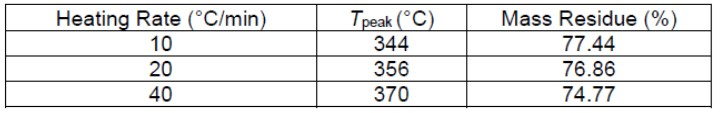The peak temperatures of DTG at each heating rate were 344, 356, and 370 ℃, respectively, shown in Fig. 1(a). The varied peak temperature was caused by thermal hysteresis. Biomass is a poor heat conductor, which leads to low heat transfer efficiency. The atmosphere temperature in TG analyzer heats up quickly with the high heating rate, and the time interval that the heat transferred to the sample center is longer, which caused the peak temperature at high heating rate to be larger, whereas a lower heating rate resulted in a uniform temperature of biomass (Loy et al. 2018).

Figure 1(b) shows that the main reaction occurred between 200 ℃ and 450 ℃. This temperature range encompasses the pyrolysis of hemicellulose, cellulose, and lignin, which are the major components of biomass. The mass residue decreased with the increase of the heating rate as shown in Table 1. The lower residual mass with higher heating rate most likely resulted from the adequate decomposition of the reactants under the high heating rate. The average final residue was 77.4%.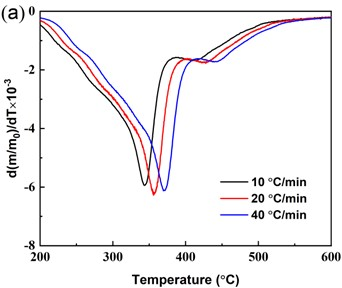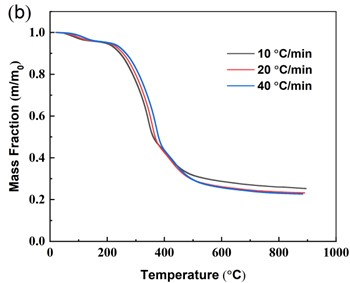Fig. 1. (a) DTG curves, (b) TG curves

Dynamic Parameter of Thermal Decomposition

The dynamic parameters of thermal decomposition can be calculated from thermogravimetric data, such as activation energy () and pre-exponential factors (A). The KAS, FWO, and K-K methods described in the Experimental section were applied to calculate the activation energy of PN and the three main constituents (hemicellulose, cellulose, and lignin).

Activation energy of PN

The conversion rate from 0.1 to 0.9 was selected to calculate the activation energy of PN at different heating rates (10, 20, and 40 ℃ /min) based on the KAS and FWO methods. As described in the Experimental section, the KAS method plots ln (β/T2) against 1/T, which was shown in Fig. 2, and the FWO method plots against 1/T, as plotted in Fig. 3. The activation energy for varied conversion rates were calculated with the slopes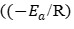of KAS and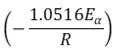of FWO). The reaction order (n) was assumed to be one, and the reaction mechanism functions were calculated as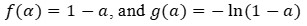. On this basis, the pre-exponential factors were also determined.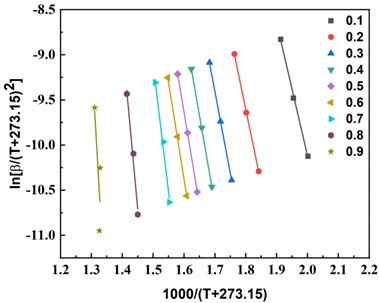Fig. 2. KAS diagram of PN at varied conversion rates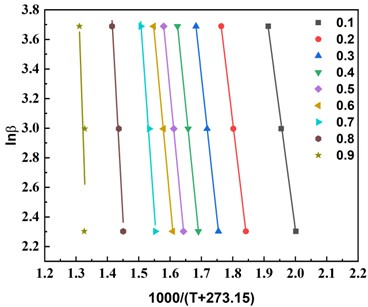Fig. 3. FWO diagram of PN at varied conversion rates

Activation energies, pre-exponential factors, and coefficients of determination (R2) of PN pyrolysis are listed in Table 2. The R2 values were high, which indicated that the results were well suitable for the model equations.

The increases with conversion rate, which denotes the decomposition of main biomass constituents. At the 0.1 < α < 0.4 stage, the activation energy range is 122 to 163 KJ/mol; this phase is mainly the hemicellulose pyrolysis stage. Decomposition takes place mainly by dehydration, decarboxylation, and the generation of small gas molecules such as decarburization and condensable volatiles, including breakage of C-O-C and C-C bonds and rupture of small molecule chains (Gangil and Bhargav 2018), which is consistent with thermogravimetric analysis. At the 0.5 to 0.7 stage, the activation energy ranged from 171 to 236 KJ/mol. This stage is mainly the pyrolysis stage of cellulose. Cellulose is a linear macromolecular polysaccharide composed of D-glucose group, which has high cohesion and hydrolysis resistance, and its degree of polymerization is higher than that of hemicelluloses (Gangil 2014). Lignin is a polymer containing an aromatic substrate that is involved in the crosslinking of hemicellulose and cellulose and thus is involved throughout the pyrolysis process. At the 0.8 to 0.9 stage, there is mainly a three-component carbonization process. The aromatic ring monomers produced by lignin depolymerization at this stage are more stable, so the activation energy is higher at this stage (Gupta et al. 2020).

The variation of indicates that there are multi-step kinetics in the pyrolysis process, and the reason for the varied activation energy is that the chemical reactions of hemicellulose, cellulose, and lignin take place mainly at different temperatures. So hemicellulose, cellulose, and lignin in PN have different activation energies.

Activation energy of main components

The degradation sequence of those main components is hemicellulose, cellulose, and lignin with required activation energy (Grønli et al. 2002). The peak temperatures of them at varied heating rates are listed in Table 3, and the |DTG| and |DDTG| curves at heating rate 10 ℃/min are shown in Fig. 4.

Table 2. Ea and A by KAS and FWO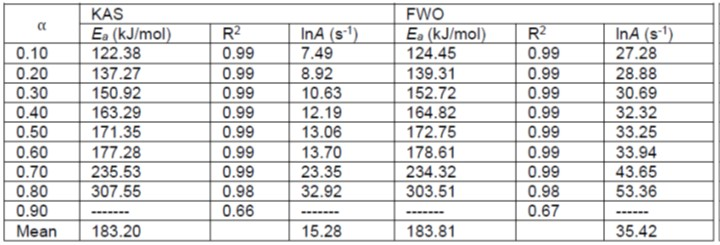Table 3. The Peak Temperature of Main Components at Different Heating Rates and Activation Energy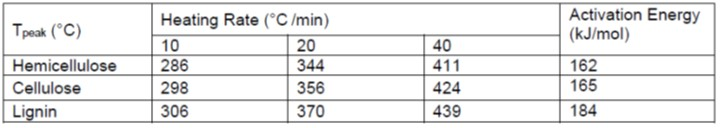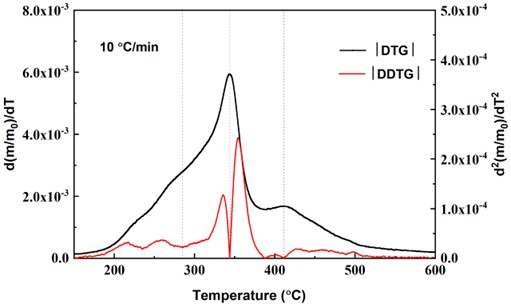Fig. 4. Estimation of the peak locations based on |DTG| and |DDTG| at 10 °C/min

The activation energy of each component was calculated with K-K method. Figure 5 shows the relationship of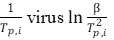. As can be seen in Table 3, the pyrolysis activation energy of the three components is in the order: lignin (184 kJ/mol) > cellulose (165 kJ/mol) > hemicellulose (162 kJ/mol). The trend was according to the activation energy of PN at varied conversion rates calculated in Table 2. Different inherent chemical structures and compositions may be caused by the varied activation energy of the main components. Hemicellulose is an amorphous structure composed of short chains with weak bonds, which is easily decomposed. Cellulose is a crystalline structure with a linear homopolysaccharide, which can be resistant to degradation. Lignin is a tridimensional structure with a basic structure unit of phenylpropane. The higher energy requirement for lignin pyrolysis is primarily attributed to its intricate and interconnected structure (Chen et al. 2022).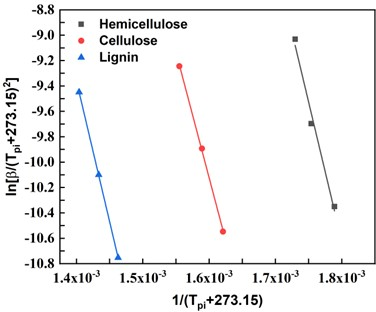Fig. 5. K-K diagram of three components of PN

TG-FTIR analysis

Figure 6 shows three-dimensional infrared spectrum of the pyrolysis volatiles of PN at 10 ℃/min, which presents the absorbance as a function of wave number and temperature, and the release characteristics of the volatile gas are basically consistent with the DTG curves.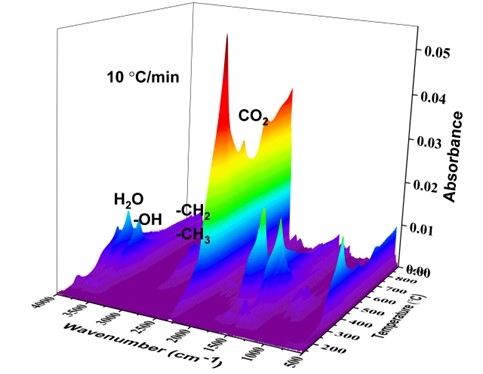Fig. 6. Three-dimensional infrared spectra of PN

The composition of the pyrolysis volatiles can be determined from the characteristic peaks of infrared spectrum and the release sequence of the products can be estimated from absorption spectrum (Ma et al. 2018). As can be seen from Fig. 4, the peak temperature during pyrolysis process of PN is 344 °C at 10 ℃/min, and the absorption spectra curve at peak temperature is shown in Fig. 7.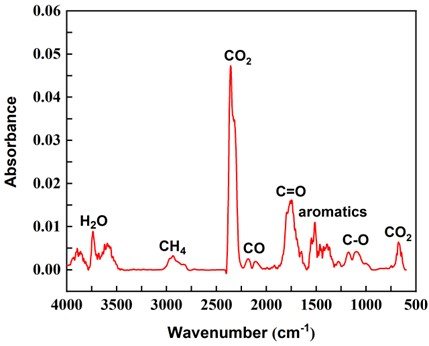Fig. 7. TG-FTIR spectra of gas emissions at the peak temperature

The absorbance bands around 3500-4000 cm-1 showed the stretching vibration of hydroxyl (-OH) in H2O. The presence of the fingerprint region 1400-1300 cm-1 represents the stretching vibration of hydroxyl group (-OH) in alcohol and phenol. The characteristic band ranged from 3000 to 2800 cm-1 and is related to C–H in methyl and methylene groups and produced CH4. The absorption peak band of 2400-2240 cm−1 is caused by C=O in carbon dioxide, and there is a bending vibration of 660-680 cm−1 of carbon dioxide in the fingerprint region. The absorption peak band of 2240-2030 cm−1 represents CO emission. The characteristic band around 1850-1600 cm-1 represents C=O containing compounds (such as acids, aldehydes, and ketones). The 1600-1450 cm−1 was generated by C=C stretching vibration in benzene rings. The absorption peak around 1400-1050 cm−1 indicates compounds containing C–O, corresponding to the presence of phenols and alcohols, while absorptions around 1380 cm−1 was assigned to C–H. The main detected pyrolysis volatiles and functional groups were H2O, CH4, CO2, CO, C=O, C=C, and C-O, which are listed in Table 4.

Table 4. Functional Groups and Gas Products of PN Pyrolysis by TG-FTIR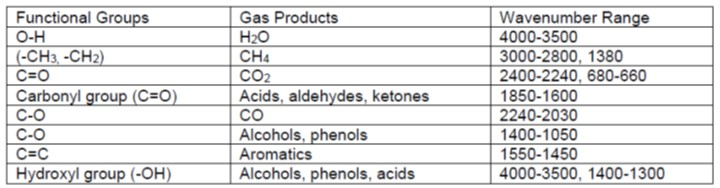According to Lambert-Beer’s Law, the absorption intensity of a specific compound becomes stronger in linear proportion as its concentration increases (Liu et al. 2008). Figure 8 presents the profiles of detected gas as the temperature increases during PN pyrolysis at a rate of 10 °C/min. Among the gases released from PN pyrolysis, the highest amount was recorded for CO2, followed by aromatic compounds and carbonyl compounds. On the other hand, CO emission was the lowest among the evolved gases. The peak temperature observed during the pyrolysis process of PN was 344 °C at a rate of 10 °C/min, which aligns with the findings from the TG experiment depicted in Fig. 4.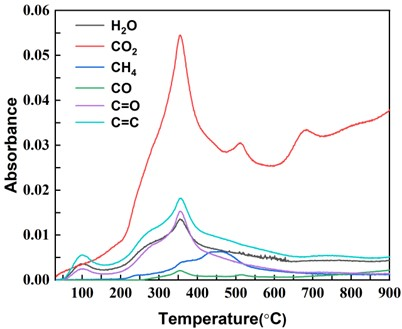Fig. 8. Peak wavelengths profiles of PN pyrolysis products at 10 °C/min

Py-GC/MS Patterns

The pyrolysis temperature plays a crucial role in determining the type and yield of product compounds (Sun et al. 2020). In this study, based on Fig. 1, it was observed that the main components of PN were mostly degraded at 600 °C. Therefore, 600°C was selected as the fast pyrolysis temperature for the Py-GC/MS. The fast pyrolysis vapor of PN consists of non-condensable gases (such as CH4, H2, CO2, CO, etc.) and volatile products. The volatile compounds undergo condensation to form liquid bio-oil, which was identified using the NIST database. The yield of different substances was estimated based on the relative peak area of each compound (Lu et al. 2011; Liu et al. 2021). Table 5 presents the main by-products of PN pyrolysis, as identified by Py-GC/MS. A total of 73 compounds were identified.

Table 5. Main By-products of the PN Pyrolysis Identified via Py-GC/MS